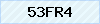### Client Registration

Client Registration

Please fill in the fields below to register for a new account. Fields marked with a * are required.

Basic Information
 +1+1 242+1 246+1 264+1 268+1 284+1 340+1 345+1 441�+1 473+1 599+1 649+1 664+1 670+1 671+1 684+1 758+1 767+1 784+1 809+1 868+1 869+1 876+20+212+213+216+218+220+221+222+223+224+225+226+227+228+229+230+231+232+233+234+235+236+237+238+239+240+241+242+243+244+245+248+249+250+251+252+253+254+255+256+257+258+260+261+262+263+264+265+266+267+268+269+27+290+291+297+298+30+31+32+33+34+350+351+352+353+354+355+356+357+358+359+36+370+371+372+373+374+375+376+377+378+380+381+382+385+386+387+389+39+40+41+420+421+423+43+44+45+46+47+48+49+500+501+502+503+504+505+506+507+508+509+51+52+53+54+55+56+57+58+590+591+592+593+595+597+598+599+60+61+62+63+64+65+66+670+672+673+674+675+676+677+678+679+680+681+682+683+685+686+687+688+689+690+691+692+7+81+82+84+850+852+853+855+856+86+870+880+886+90+91+92+93+94+95+960+961+962+963+964+965+966+967+968+970+971+972+973+974+975+976+977+98+992+993+994+995+996+998
 +1+1 242+1 246+1 264+1 268+1 284+1 340+1 345+1 441�+1 473+1 599+1 649+1 664+1 670+1 671+1 684+1 758+1 767+1 784+1 809+1 868+1 869+1 876+20+212+213+216+218+220+221+222+223+224+225+226+227+228+229+230+231+232+233+234+235+236+237+238+239+240+241+242+243+244+245+248+249+250+251+252+253+254+255+256+257+258+260+261+262+263+264+265+266+267+268+269+27+290+291+297+298+30+31+32+33+34+350+351+352+353+354+355+356+357+358+359+36+370+371+372+373+374+375+376+377+378+380+381+382+385+386+387+389+39+40+41+420+421+423+43+44+45+46+47+48+49+500+501+502+503+504+505+506+507+508+509+51+52+53+54+55+56+57+58+590+591+592+593+595+597+598+599+60+61+62+63+64+65+66+670+672+673+674+675+676+677+678+679+680+681+682+683+685+686+687+688+689+690+691+692+7+81+82+84+850+852+853+855+856+86+870+880+886+90+91+92+93+94+95+960+961+962+963+964+965+966+967+968+970+971+972+973+974+975+976+977+98+992+993+994+995+996+998

Note: Email Address will be used to sign-in to your account and for all future communication with us. Secondary Email Address will be used in case if your primary email address is non operational or if you forget your password